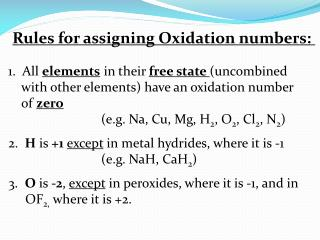DownloadDownload PresentationRules for assigning Oxidation numbers:

# Rules for assigning Oxidation numbers:

Télécharger la présentation## Rules for assigning Oxidation numbers:

- - - - - - - - - - - - - - - - - - - - - - - - - - - E N D - - - - - - - - - - - - - - - - - - - - - - - - - - -
##### Presentation Transcript

1. Rules for assigning Oxidation numbers: 1. All elements in their free state (uncombined with other elements) have an oxidation number of zero (e.g. Na, Cu, Mg, H2, O2, Cl2, N2) 2. His +1except in metal hydrides, where it is -1 (e.g. NaH, CaH2) 3. Ois -2, except in peroxides, where it is -1, and in OF2, where it is +2.

2. 4. The metallic element in an ioniccompound has a positive oxidation number 5. In covalent compounds the negativeoxidation number is assigned to the most electronegative atom. 6. The algebraic sum of the oxidation numbers of the elements in a compound is zero. 7. The algebraic sum of the oxidation numbers of the elements in a polyatomic ion is equal to the chargeof the ion.

3. Determine the oxidation number of (a) S in Na2SO4, (b) As in K3AsO4, and (c) C in CaCO3 K3 (AsO4)3- Na2 (SO4)2- Ca (CO3)2- 1+ 5+ 2- 1+ 6+ 2- 2+ 4+ 2-

4. Determine the oxidation numbers of (a) N in NH4+, (b) Cr in (Cr2O7)2- and (c) P in (PO4)3- (Cr2O7)2- (NH4)+ (PO4)3- 6+ 2- • 3- • 1+ 5+ 2-

5. Determine the oxidation number of each element in these species: BaCl2 H(ClO) 2+ 1- 1+ 1+ 2- H2O2 (peroxide) 1+ 1- (BrO3)- 5+ 2-

6. Oxidation: Increase in oxidation number (loss of e-) Reduction: Decrease in oxidation number (gain of e-) OILRIG LEOGER

7. Oxidizing agent (is reduced, gains e-) – the substance that causes an increase in the oxidation state of another substance Reducing agent (is oxidized, loses e-) – the substance that causes a decrease in the oxidation state of another substance

8. 1+ 1- 1- 0 2+ 0 Cu + 2 AgNO3 → Cu(NO3)2 + 2 Ag RA OA • Remember: • -only reactants are oxidized or reduced “Half Reaction” - shows only one half of the redox (Ox) Cu → Cu2++ 2e- 2e- + 2Ag+ → 2 Ag (Red)